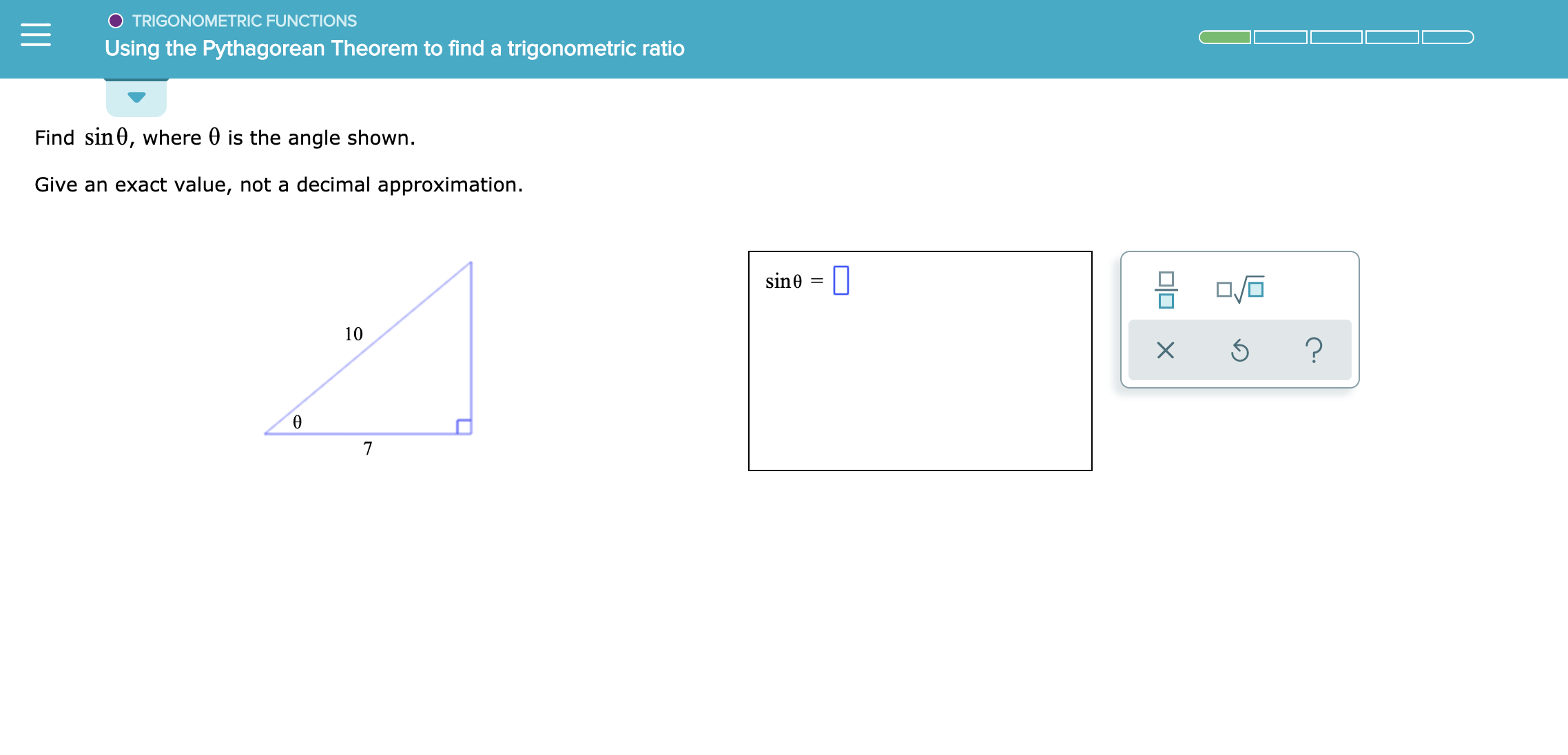# O TRIGONOMETRIC FUNCTIONSUsing the Pythagorean Theorem to find a trigonometric ratioFind sin0, where 0 is the angle shownGive an exact value, not a decimal approximationsin0| |10?Ө7미□ ×

Question
1 views

See attachmenthelp_outlineImage TranscriptioncloseO TRIGONOMETRIC FUNCTIONS Using the Pythagorean Theorem to find a trigonometric ratio Find sin0, where 0 is the angle shown Give an exact value, not a decimal approximation sin0| | 10 ? Ө 7 미□ × fullscreen
check_circle

Step 1

As,

Step 2

Side in front of angle is perpendicular line. As shown below

Step 3

Given,

Base(B) = 7 and Perpendicular(P) = 10...

### Want to see the full answer?

See Solution

#### Want to see this answer and more?

Solutions are written by subject experts who are available 24/7. Questions are typically answered within 1 hour.*

See Solution
*Response times may vary by subject and question.
Tagged in
MathCalculus

### Other